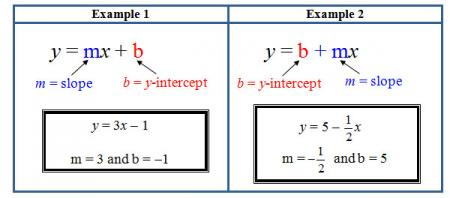# Let's Get Started

Let's explore the effects that slope and the y-intercept have on the graph. You will discover how the graph changes when the slope and y-intercept change.

TEKS Standards and Student Expectations

A(3) Linear functions, equations, and inequalities. The student applies the mathematical process standards when using graphs of linear functions, key features, and related transformations to represent in multiple ways and solve, with and without technology, equations, inequalities, and systems of equations. The student is expected to:

A(3)(E)  determine the effects on the graph of the parent function f(x) = x when f(x) is replaced by af(x), f(x) + d, f(x - c), f(bx) for specific values of a, b, c, and d

Resource Objective(s)

Given algebraic, graphical, or verbal representations of linear functions, the student will determine the effects on the graph of the parent function, f(x) = x.

Essential Questions

How does the graph change when the slope becomes negative?

How does the graph change when the slope increases?

How does the graph change when the y-intercept changes?

Vocabulary

# Slope and y-intercept

Before beginning this lesson, you need to recall how to find the slope and y-intercept when given an equation that is solved for y. The following table shows two examples. Study the examples closely before moving on.If you need more help finding the slope and y-intercept of a line given the equation, watch the video below.

Video Segment:  Find the Slope and y-intercept from an Equation

Source
Find the Slope and y-intercept from Equation, APUS07, YouTube

# The Effects of Changing Slope—Interactive Applet and Instructional Video

A linear function has two parameters that can be changed: slope (m) and y-intercept (b).  You can access an interactive applet to investigate the effect of changing the parameters on a linear function.

Directions:

1. Move the sliders to change the slope and y-intercept for the linear function.
2. Observe what happens to the graph as the parameter values change.

View this instructional video to study the effects of changing the slope and y-intercept on a linear function.

Source

# Graphing Calculator Activity 1: Shifting Lines

Complete the table below on your own paper.  Then graph the lines on the same screen using a graphing calculator. You can use a free online graphing calculator if necessary by clicking hereOnce you are finished, click on the plus sign to check your answers.

Notice any similarities and differences of the graphs of these lines.

# Graphing Calculator Activity 2: Hitting the Slopes

Complete the table below on your own paper.  Then graph the lines on the same screen using a graphing calculator. You can use a free online graphing calculator if necessary by clicking here. Once you are finished, click on the plus sign to check your answers.

Describe the similarities and differences of the graphs of these lines in activity 2.